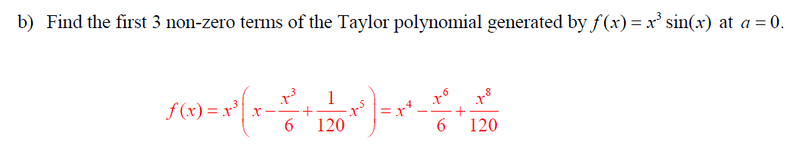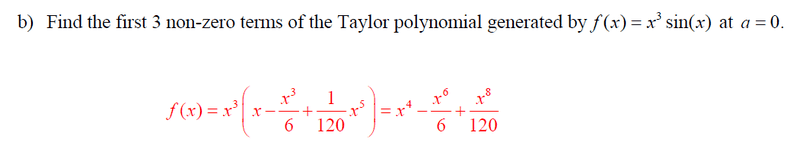# Taylor series

## Homework Statement

Find the first 3 non-zero terms of the Taylor polynomial generated by f (x) = $x^{3}$ sin(x) at a = 0.

## Homework Equations

$f^{n}$(x) * $(x-a)^{n}$ / (n!)

## The Attempt at a Solution

I got the question wrong: my answer was 1/3! + 1/5! + 1/7!
Here is the answer below. I was wondering how my teacher got that answer. Do you have to do the product rule to find the derivatives?LCKurtz
Homework Helper
Gold Member
Do you know the Taylor series for ##\sin x\ ##? He just multiplied that by ##x^3##.

Mark44
Mentor

## Homework Statement

Find the first 3 non-zero terms of the Taylor polynomial generated by f (x) = $x^{3}$ sin(x) at a = 0.

## Homework Equations

$f^{n}$(x) * $(x-a)^{n}$ / (n!)

## The Attempt at a Solution

I got the question wrong: my answer was 1/3! + 1/5! + 1/7!
Here is the answer below. I was wondering how my teacher got that answer. Do you have to do the product rule to find the derivatives?The Taylor polynomial is a function of x. Your answer is really just a single number.

You wrote something in the relevant equation section. Do you know what it represents and what it's used for?

Your textbook undoubtedly has some examples that are like this problem. Have you looked at any of them?

DryRun
Gold Member
At a=0, it means that the function f(x) is approximated near the point x=a=0. In other words, you have a Maclaurin series. Adapt and use the relevant equation from your post #1.